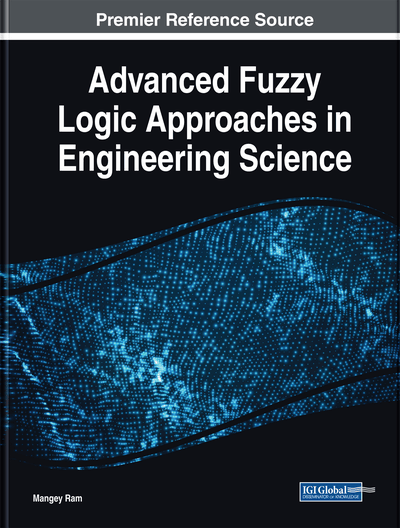# Fuzzy Sumudu Transform Approach to Solving Fuzzy Differential Equations With Z-Numbers

Raheleh Jafari (Agder University College – Grimstad, Norway), Sina Razvarz (National Polytechnic Institute, Mexico), Alexander Gegov (University of Portsmouth, UK), Satyam Paul (National Polytechnic Institute, Mexico), and Sajjad Keshtkar (Universidad Nacional Autonoma de Mexico (UNAM), Mexico)
DOI: 10.4018/978-1-5225-5709-8.ch002
Available
\$29.50
No Current Special Offers

## Abstract

Uncertain nonlinear systems can be modeled with fuzzy differential equations (FDEs) and the solutions of these equations are applied to analyze many engineering problems. However, it is very difficult to obtain solutions of FDEs. In this book chapter, the solutions of FDEs are approximated by utilizing the fuzzy Sumudu transform (FST) method. Here, the uncertainties are in the sense of fuzzy numbers and Z-numbers. Important theorems are laid down to illustrate the properties of FST. This new technique is compared with Average Euler method and Max-Min Euler method. The theoretical analysis and simulation results show that the FST method is effective in estimating the solutions of FDEs.
Chapter Preview
Top

## Introduction

In many physical and dynamical processes, mathematical modeling leads to the deterministic initial and boundary value problems. In practical, the boundary values may be different from crisp and displays in the form of unknown parameters (Zwillinger, 1998). When the parameters or the states of the differential equations are uncertain, they can be modeled with FDE. The fuzzy derivative and FDE have been studied in (Buckley, Eslami, & Feuring, 2002; Diamond & Kloeden, 1994). In recent days, many methods have used FDE for modeling and control of uncertain nonlinear systems (Jafari & Yu, 2015a, 2015b, 2017a, 2017b; Jafari, Yu, & Li, 2016). The basic idea of the fuzzy derivative was first introduced in (Chang & Zadeh, 1972). Then it is extended in (Dubois & Prade, 1982). The Peano-like theorems for FDEs and system of FDE on R (Real line) are demonstrated in (Kloeden, 1991). The application of the numerical method in order to solve FDEs has been mentioned in (Friedman, Ming, & Kandel, 1999). By generalizing the differentiability, (Bede, Rudas, & Bencsik, 2007) gave an analytical solution. The Lipschitz condition, as well as the theorem for existence and uniqueness of the solution related to FDEs, are discussed in (Allahviranloo & Ahmadi, 2010; Ding, Ma, & Kandel, 1997; Truong, Ngo, & Nguyen, 2013). In (Allahviranloo, Kiani, & Barkhor, 2009), the analytical solutions of second order FDE are obtained. The analytical solutions of third order linear FDE are found in (Hawraa & Amal, 2013). By the interval-valued method, (Stefanini, 2009) examined the basic solutions of nonlinear FDEs with generalized differentiability. The fractional fuzzy Laplace transformation has been discussed in (Allahviranloo & Ahmadi, 2010).

A novel technique in order to solve FDEs is laid down based on the Sumudu transform. Sumudu transform along with broad applications has been utilized in the area of system engineering and applied physics (Belgacem, Karaballi, & Kalla, 2003; Liu & Chen, 2016; Srivastava, Khalili Golmankhaneh, Baleanu, & Yang, 2014). In (Belgacem & Karaballi, 2006), some simple and deeper fundamental theorems, as well as properties of the Sumudu Transform, were generalized. In (Kiliman, Eltayeb, & Agrwal, 2010), Sumudu transform is applied to the system of differential equations. In (Abdul Rahman & Ahmad, 2016), Sumudu transform is used in order to find the solution of the fuzzy partial differential equation. In (Katatbeh & Belgacem, 2011), Sumudu transform has been used to solve fractional differential equations. In (Liu & Chen, 2016) the variational iteration method is suggested by means of the Sumudu transform in order to resolve ordinary equations.

## Complete Chapter List

Search this Book:
Reset